# Facebook Data Scientist Interview Question and Solution in SQL - Post Success Rate

This interview question for a data scientist position was asked by Facebook during a data science interview.

The article covers both the question and the answer and gives a detailed explanation of the approach I take to answer this Facebook data scientist interview question. I walk through each step of my answer, assumptions, approach, and explain every line of code I write.

### Question

Our suggestion is to try to solve this Facebook data scientist interview question first before reading the solution. You can try to solve the interview question here (https://platform.stratascratch.com/edu-content-editor?id=10286&python=).

#### Write a query to get the post success rate by date.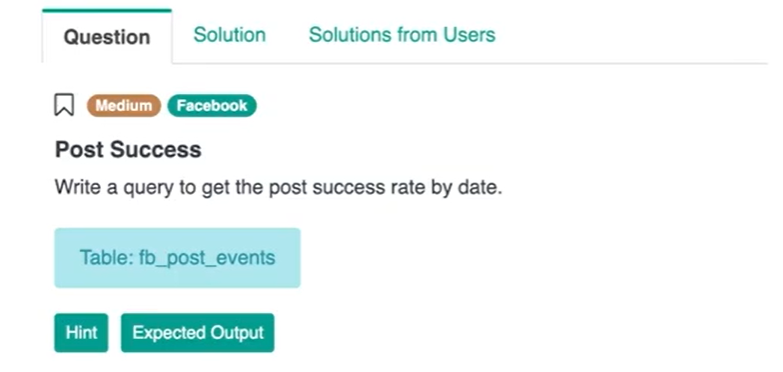The Facebook data scientist interview question asks the interviewee to write a query to get the post success rate by date. Technically, this question leverages SQL concepts like CASE WHEN and SUBQUERIES. This question covers concepts that are commonly found in data science interviews at Facebook and Google.

You're getting this question during a Facebook data science interview and you are given the table schema, and the table is called Facebook post events. We have a user_id, a created_at which is probably date and the event_name. If we preview this data, we get a record of user_id, date and then an action event_name.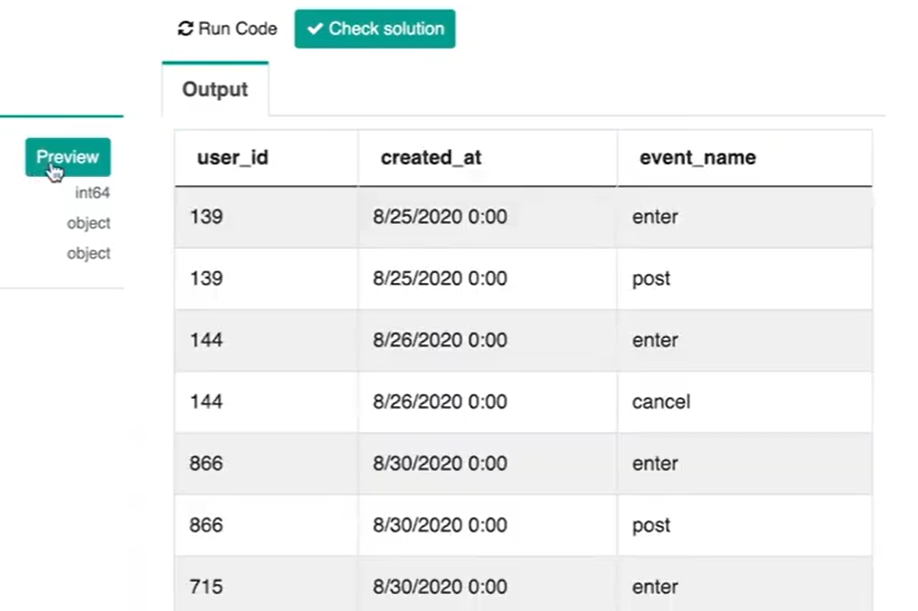### Assumptions

My assumption is that for every post I'll first have an ‘enter’ as an event_name. So, if the user is trying to post either a picture or a message or something, the first thing that gets logged by the platform is this event_name ‘enter’.
If it's successful the post will be called ‘post’. That is a successful post. If the post is not successful then you'll see this event name as ‘cancel’.

### Approach

#### Defining post success rate

What we need to do to get this post success rate is we define that as the number of ‘posts’ divided by the number of ‘enters’ that we see in the data.

``post success rate = # post / # enter``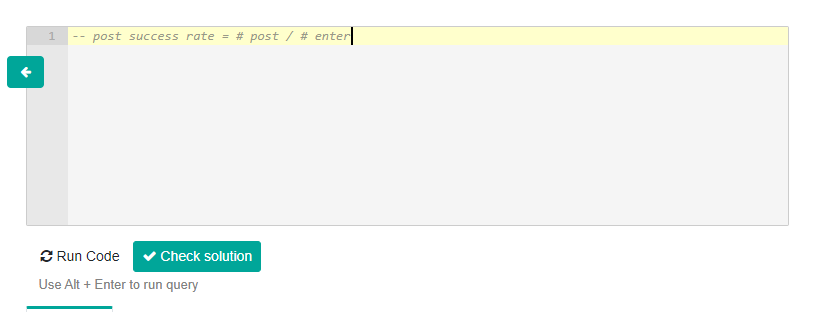#### Creating a query

The next thing I should do is creating a query that counts the number of ‘enters’ and then counts the number of ‘posts’ and also counts the number of ‘cancels’. That's going to look like:

``````SELECT
created_at
, count(CASE WHEN event_name = 'enter' THEN user_id END) as enter
, count(CASE WHEN event_name = 'cancel' THEN user_id END) as cancel
, count(CASE WHEN event_name = 'post' THEN user_id END) as post
FROM fb_post_events
GROUP BY created_at``````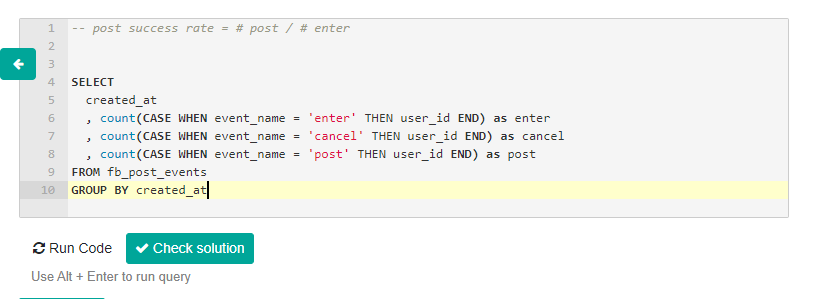If I just want to see whether or not my code was written correctly, I can run this block of code and I see that I am able to capture and count the number of ‘enters’, ‘cancels’ and ‘posts’ by date.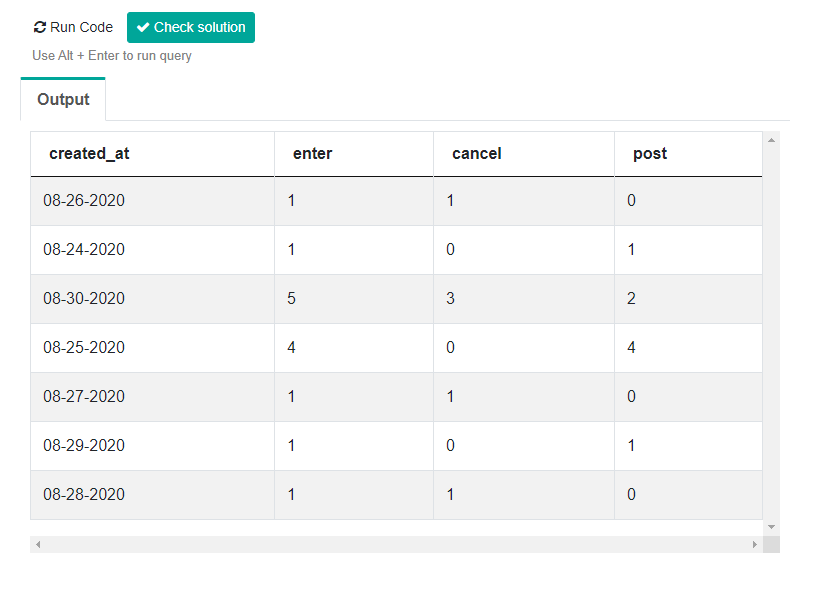#### Creating the metric

Now, all I need to do is create the metric. I'm going to use what I just wrote as a subquery to another query that's going to be doing this operation. Below is what it's going to look like:

``````SELECT
created_at,
post/enter::float as post_success_rate
FROM (
SELECT
created_at
, count(CASE WHEN event_name = 'enter' THEN user_id END) as enter
, count(CASE WHEN event_name = 'cancel' THEN user_id END) as cancel
, count(CASE WHEN event_name = 'post' THEN user_id END) as post
FROM fb_post_events
GROUP BY created_at) a
GROUP BY created_at``````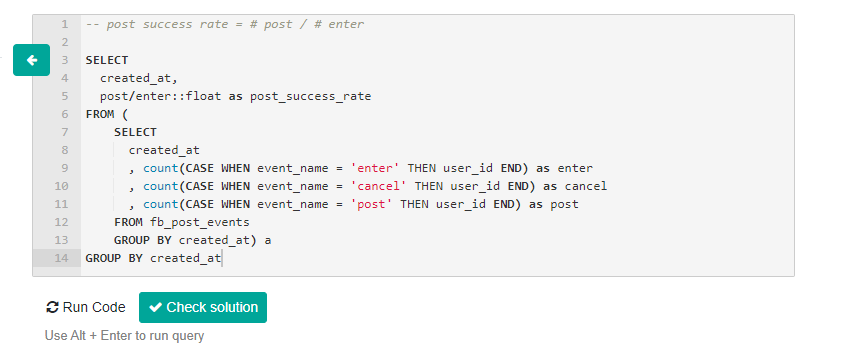So, it's a post success rate by date. You can see the date field and the operation where I have a count of ‘posts’. I'm dividing that by the count of ‘enters’ and I'm actually changing the data type to a FLOAT because the ‘post’ is an integer and the ‘enter’ is also an integer. And an integer divided by an integer will always output as an integer. So, it's going to be a 1 unless I'm changing this data type to a FLOAT.

### Solution

What I want to add to this query is the count of ‘posts’ as well as ‘enters’ so that I have just the number to quality check whether or not this rate is calculated correctly. If I add all of the columns to the GROUP BY and press run code what I do get is the post success rate.

``````SELECT
created_at,
post,
enter,
post/enter::float as post_success_rate
FROM (
SELECT
created_at
, count(CASE WHEN event_name = 'enter' THEN user_id END) as enter
, count(CASE WHEN event_name = 'cancel' THEN user_id END) as cancel
, count(CASE WHEN event_name = 'post' THEN user_id END) as post
FROM fb_post_events
GROUP BY created_at) a
GROUP BY created_at, post, enter``````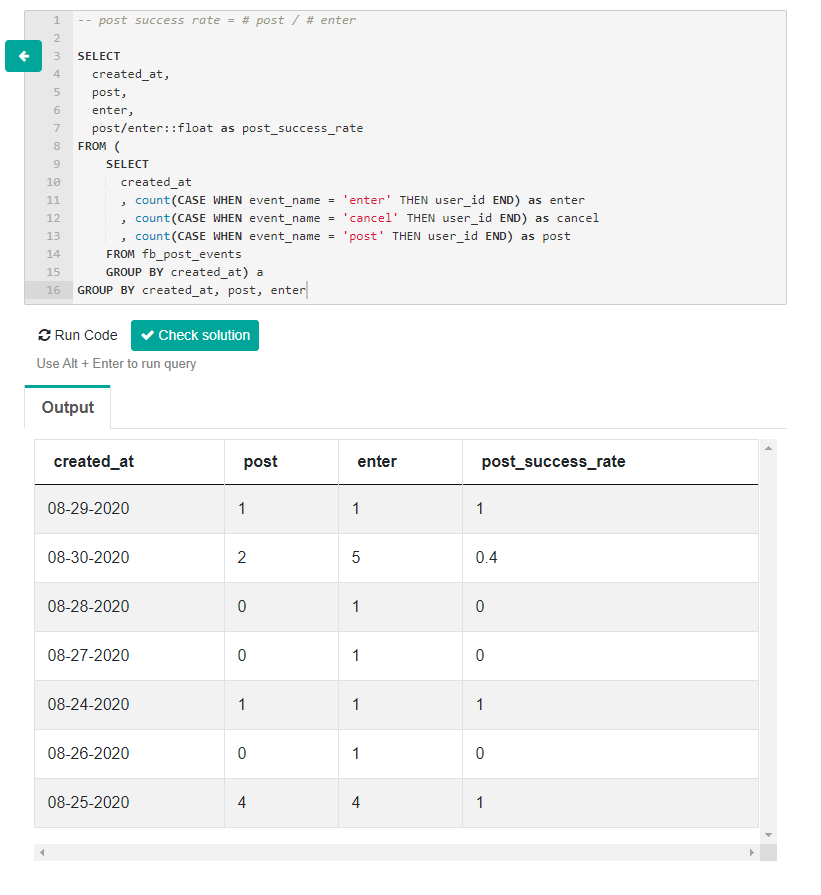So, 1 equals 100 percent and 0.4 should equal 40 percent. If I add the columns in the GROUP BY as I've done already and then press run code, what we get is the date, the count on posts for that date, the count on the number of enters for that date, and then the post success rate. So, 1 equals 100 percent, 0 is 0 percent, and 0.4 is 40 percent.

Strata Scratch, LLC © 2021
team@stratascratch.com